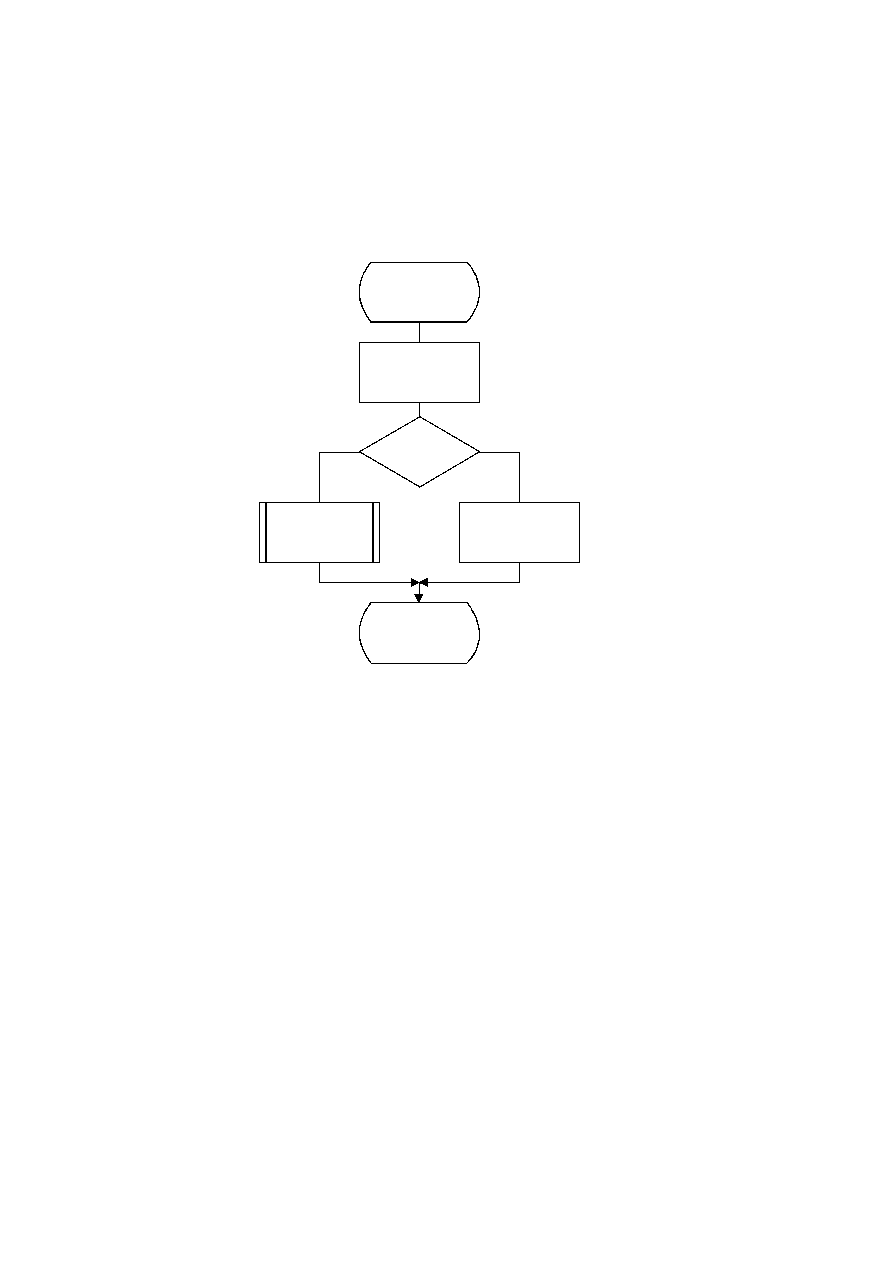ISO/IEC 10918-1 : 1993(E)
The Byte_in procedure used in Renorm_d is shown in Figure D.20. This procedure fetches one byte of data,
compensating for the stuffed zero byte which follows any X'FF' byte. It also detects the marker which must follow the
entropy-coded segment. The C-register in this procedure is the concatenation of the Cx and C-low registers. For simplicity
of exposition, the buffer holding the entropy-coded segment is assumed to be large enough to contain the entire segment.
B is the byte pointed to by the entropy-coded segment pointer BP. BP is first incremented. If the new value of B is not a
X'FF', it is inserted into the high order 8 bits of C-low.
TISO1200-93/d058
Byte_in
BP = BP + 1
Yes
No
Unstuff_0
C = C + SLL B 8
Done
B = X'FF'
?
Figure D.20 ­ Byte_in procedure for decoder
Figure D.20 [D58], = 12 cm = 469.%
74
CCITT Rec. T.81 (1992 E)